JEE  >  Test: Introduction To Vector Algebra

# Test: Introduction To Vector Algebra - JEE

Test Description

## 20 Questions MCQ Test Mathematics (Maths) Class 12 - Test: Introduction To Vector Algebra

Test: Introduction To Vector Algebra for JEE 2023 is part of Mathematics (Maths) Class 12 preparation. The Test: Introduction To Vector Algebra questions and answers have been prepared according to the JEE exam syllabus.The Test: Introduction To Vector Algebra MCQs are made for JEE 2023 Exam. Find important definitions, questions, notes, meanings, examples, exercises, MCQs and online tests for Test: Introduction To Vector Algebra below.
Solutions of Test: Introduction To Vector Algebra questions in English are available as part of our Mathematics (Maths) Class 12 for JEE & Test: Introduction To Vector Algebra solutions in Hindi for Mathematics (Maths) Class 12 course. Download more important topics, notes, lectures and mock test series for JEE Exam by signing up for free. Attempt Test: Introduction To Vector Algebra | 20 questions in 20 minutes | Mock test for JEE preparation | Free important questions MCQ to study Mathematics (Maths) Class 12 for JEE Exam | Download free PDF with solutions
 1 Crore+ students have signed up on EduRev. Have you?
Test: Introduction To Vector Algebra - Question 1

### For what values of x and y, the vectors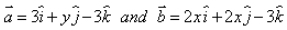are equal?

Detailed Solution for Test: Introduction To Vector Algebra - Question 1

Two vectors are equal if their corresponding components are equal.
Hence, 2x = 3
or x = 3/2
and 2x = y
or y = 2 * 3/2
y = 3

Test: Introduction To Vector Algebra - Question 2

### Two or more vectors having the same initial point are called​

Detailed Solution for Test: Introduction To Vector Algebra - Question 2

Two or more vectors having the same initial point are called coinitial vectors.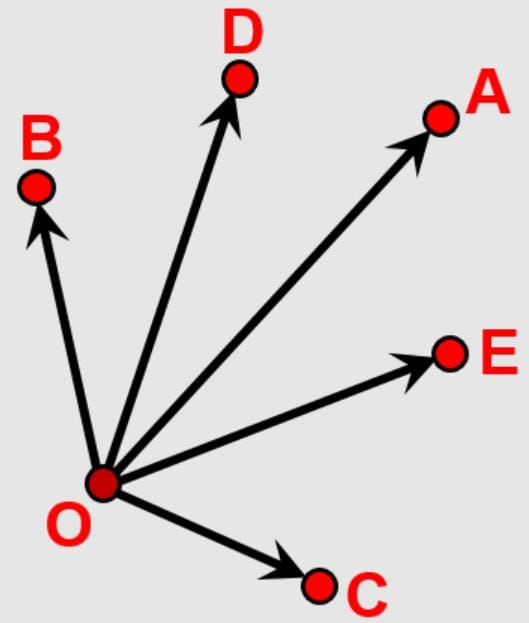Test: Introduction To Vector Algebra - Question 3

### If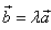,the vectors a and b are ______ .​

Detailed Solution for Test: Introduction To Vector Algebra - Question 3Both of these vectors are pointing the same direction but they have different magnitude. Hence, they are collinear vectors.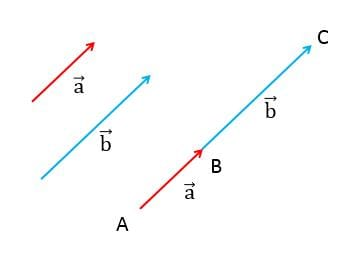Test: Introduction To Vector Algebra - Question 4

If the magnitude of the position vector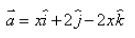is 7, the value of x is:​

Detailed Solution for Test: Introduction To Vector Algebra - Question 4|a| = (x2 + 22 + (2x)2)1/2
7 = (x2 + 22 + (2x)2)1/2
⇒ 49 = x2 + 22 + 4x2
⇒ 49 = 4 + 5x2
⇒ 5x2 = 45
⇒ x2 = 9
x = ±3

Test: Introduction To Vector Algebra - Question 5

If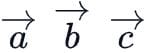and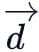are the position vectors of the points A, B, C and D such that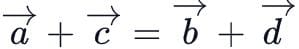then ABCD is:

Detailed Solution for Test: Introduction To Vector Algebra - Question 5

Given: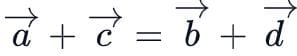If we divide both sides with 2 we get,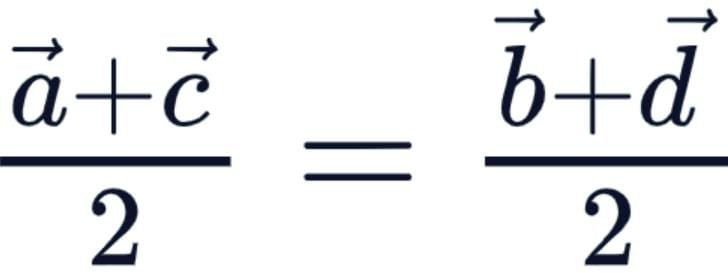⇒ Mid pt. of AC = Mid. pt. of BD
∴ ABCD is a parallelogram.

Test: Introduction To Vector Algebra - Question 6

If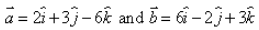, then

Detailed Solution for Test: Introduction To Vector Algebra - Question 6

a = 2i + 3j - 6k
|a| = √4+9+36 = √49 = 7
b = 6i - 2j + 3k
|b| = √36+4+9 = √49 = 7
|a| = |b|
Hence, option A is correct.

Test: Introduction To Vector Algebra - Question 7

What is the additive identity of a vector?​

Detailed Solution for Test: Introduction To Vector Algebra - Question 7

In the Additive Identity of vectors, the additive identity is zero vector 0.
For any vector V additive identity is defined as,
0 + V = V and V + 0 = V

Test: Introduction To Vector Algebra - Question 8

The angles α, β, γ made by the vector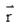with the positive directions of X, Y and Z-axes respectively, then the direction cosines of the vectorare:

Detailed Solution for Test: Introduction To Vector Algebra - Question 8

Direction Cosines for angle α, β, γ are:
cos α, cos β, cos γ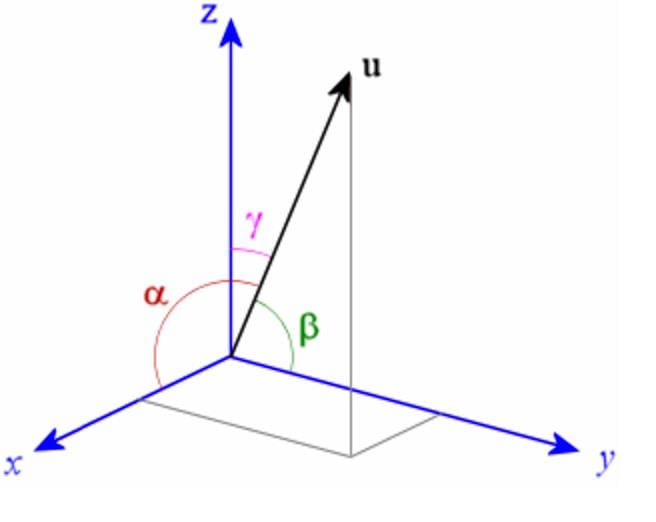Test: Introduction To Vector Algebra - Question 9

If a and b are the position vectors of two points A and B and C is a point on AB produced such that AC = 3AB, then position vector of C will be​

Detailed Solution for Test: Introduction To Vector Algebra - Question 9

Position vector of A = OA = a
Position vector of B = OB = b
Now, AC = OC - OA
Also, AB = OB - OA
Given, AC = 3AB
⇒ OC - OA = 3(OB - OA)
⇒ OC = 3b - 2a

Test: Introduction To Vector Algebra - Question 10

Vector of magnitude 1 is called.​

Detailed Solution for Test: Introduction To Vector Algebra - Question 10

A vector whose magnitude (i.e., length) is equal to 1 is called a unit vector. There are exactly two unit vectors in any given direction and one is the negative of the other.

Test: Introduction To Vector Algebra - Question 11

If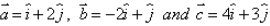and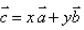, then the value of scalars x and y are:

Detailed Solution for Test: Introduction To Vector Algebra - Question 11

Given, a = i + 2j
b = -2i + j
c = 4i +3j
Also, c = xa +yb
Now putting the values in above equation,
4i + 3j  = x(i + 2j) + y(-2i +j)
⇒ xi + 2xj - 2yi + yj
⇒ (x-2y)i + (2x+y)j
We get,
x - 2y = 4
2x + y = 3
After solving,
x = 2
y = -1

Test: Introduction To Vector Algebra - Question 12

The direction of zero vector.​

Detailed Solution for Test: Introduction To Vector Algebra - Question 12

Zero vector is the unit vector having zero length, hence the direction is undefined.

Test: Introduction To Vector Algebra - Question 13

The unit vector in the direction of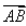, where A and B are the points (2, – 3, 7) and (1, 3, – 4) is:

Detailed Solution for Test: Introduction To Vector Algebra - Question 13

Given, Point A (2,-3,7)

Point B (1,3,-4)

Let vector in the direction of AB be C.

∴ C = B - A

⇒ (1,3,-4) - (2,-3,7)

⇒ ( 1-2 , 3+3 , -4-7 )

⇒ (-1,6,-11)

⇒ -1i + 6j -11k
Magnitude of vector C
|C| = √(-1)2 + 62 + (-11)2
⇒ √1+36+121
⇒ √158

Unit vector = (Vector)/(Magnitude of vector)
Unit vector C = (C vector)/(Magnitude of C vector)  = (-1i + 6j -11k)/√158

Test: Introduction To Vector Algebra - Question 14

If a be magnitude of vector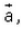then​

Detailed Solution for Test: Introduction To Vector Algebra - Question 14

Since a is the magnitude of the vector, it is always positive and it can be 0 in case of zero vectors.
So, a ≥ 0

Test: Introduction To Vector Algebra - Question 15

A vector of magnitude 14 units, which is parallel to the vector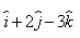Detailed Solution for Test: Introduction To Vector Algebra - Question 15

Given vector = i + 2j - 3k
Magnitude = √12 + 22 + (-3)2 = √14
Unit vector in direction of resultant = (i + 2j - 3k) / √14
Vector of magnitude 14​ unit in direction of resultant,
⇒ 14[ (i + 2j - 3k) / √14 ]
⇒ √14(i + 2j - 3k)

Test: Introduction To Vector Algebra - Question 16

For any two vectors a and b​, we always have

Detailed Solution for Test: Introduction To Vector Algebra - Question 16

|a + b|2 = |a|2 + |b|2 + 2|a||b|.cosθ
|a|2 + |b|2 = |a|2 + |b|2 + 2|a| + |b|  ∵ −1 ⩽ cosθ ⩽ 1
⇒ 2|a||b|.cosθ ⩽ 2|a||b|
So, |a + b|2 ⩽ (|a| + |b|)2
⇒ |a + b| ≤ |a| + |b|
This is also known as Triangle Inequality of vectors.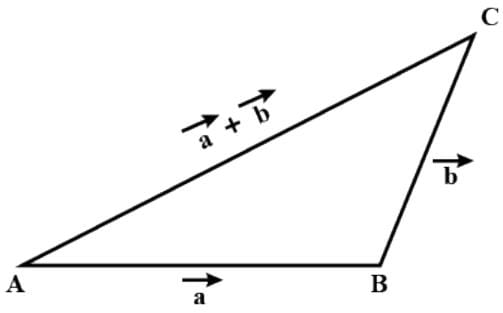Test: Introduction To Vector Algebra - Question 17

If l, m, n are the direction cosines of a position vector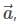then which of the following is true?

Detailed Solution for Test: Introduction To Vector Algebra - Question 17

Consideris the position vector of a point M(x,y,z) and α, β, γ are the angles, made by the vectorwith the positive directions of x, y and z respectively. The cosines of the angles, cos⁡α, cos⁡β, cos⁡γ are the direction cosines of the vectordenoted by l, m, n, then
cos2⁡α + cos2⁡β+ cos2⁡γ =1  i.e.l+ m+ n= 1.

Test: Introduction To Vector Algebra - Question 18

A vector whose initial and terminal points coincide, is called​

Detailed Solution for Test: Introduction To Vector Algebra - Question 18

A vector whose initial and terminal points coincide has no particular direction and 0 magnitude. Therefore, it is called zero vector.

Test: Introduction To Vector Algebra - Question 19

A point from a vector starts is called______and where it ends is called its______.​

Detailed Solution for Test: Introduction To Vector Algebra - Question 19

A vector is a specific quantity drawn as a line segment with an arrowhead at one end. It has an initial point, where it begins, and a terminal point, where it ends. A vector is defined by its magnitude, or the length of the line, and its direction, indicated by an arrowhead at the terminal point.

Test: Introduction To Vector Algebra - Question 20

If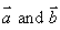are position vectors of the points (- 1, 1) and (m, – 2). then for what value of m, the vectorsare collinear.

Detailed Solution for Test: Introduction To Vector Algebra - Question 20

Given a = (-1,1) and b = (m,-2)
Given that above two vectors are collinear, so they are parallel
⇒ -1/m = 1/-2
⇒ m = 2

## Mathematics (Maths) Class 12

206 videos|264 docs|139 tests
Information about Test: Introduction To Vector Algebra Page
In this test you can find the Exam questions for Test: Introduction To Vector Algebra solved & explained in the simplest way possible. Besides giving Questions and answers for Test: Introduction To Vector Algebra , EduRev gives you an ample number of Online tests for practice

## Mathematics (Maths) Class 12

206 videos|264 docs|139 tests